# Behind the Scenes of Digital Image with Python

Back when photography and patience mattered to widely respected landscape photographer Ansel Eaton Adams, it was almost impossible to fathom the possibility of digital images. His masterpieces did not require any touchups or alteration. However, it took him decades to perfect his pure photography technique with great technical details.

In this generation of digital images, photoshopping or image processing saves photographers time and effort to perfect the image. We use image processing software such as Adobe Photoshop and GNU Image Manipulation Program to fix imperfections, save damaged photos, create out of this world art, etc. To demonstrate, you can see that the two images below are a side by side comparison of the original photo and the processed photo.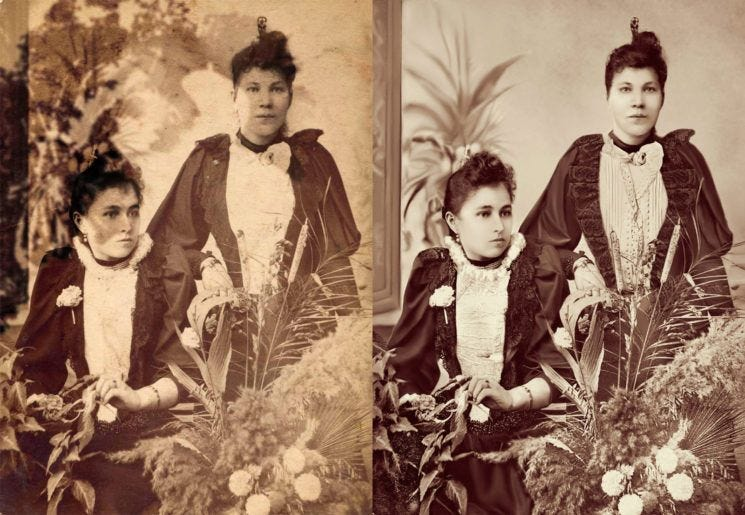Left: Double exposed original image; Right: Processed image.

If you pay attention to the lace details in both photos, you would wonder how a processed photo would have sharper details than the original? How was the background noise in the left image removed? How is it even possible that we can alter digital images?

Let’s step back a bit. A DIGITAL IMAGE IS A COLLECTION OF PIXELS. When you put together several pixels, you can probably make a simple digital image of Mario.

Let’s use the mathematical form of pixels to understand digital images. We can use several Python libraries to handle image processing in digital images. Let’s begin with the basic NumPy and Scikit-image (or skimage) as shown in the first code snippet.

`import numpy as npfrom skimage.io import imshow, imread`

We can represent a grayscale image with a two-dimensional matrix of values, which are the pixels. We chose the numbers 0 and 255 because the number 0 represents the black shade, and the number 255 represents the white shade. Any number between 0 and 255 will have a gray shade. Moreover, we can use the np.array line of code to create the matrix called “array” and use the imshow() function to display the image form of the “array.”

`array = np.array([[255, 0],                  [0, 255])imshow(array, cmap='gray');`

This image has four pixels where the upper left and bottom right are white, and the upper right and bottom left are black.

Let’s try to use a colored photo to show how the pixels or matrices are like. First, we have to remember that colored photos are represented by combinations of the three primary colors — red, green, blue. Since three colors are involved, the matrices will be three-dimensional. As you can check in the code snippet below, the color_array has three values per list. For example, the upper-left pixel in the colored photo below is colored pink, represented by 255 as the red channel's value, 192 as the green channel’s value, and 203 as the blue channel's value.

`color_array = np.array([[[255, 192, 203],                       [0, 245, 249]],                       [[48, 349, 190],                       [45, 67, 190]]])imshow(array);`

These two samples have shown that any digital image can be represented in matrice form. Let’s try using a high-resolution image of a girl.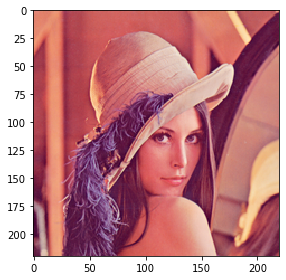A colored image of a girl
`lena = imread('lena.png')lena#Result of the lena arrayarray([[[225, 137, 127],        [224, 137, 127],        [227, 134, 119],        ...,        [227, 141, 128],        [232, 150, 124],        [213, 120, 104]],       [[225, 137, 127],        [224, 136, 127],        [227, 134, 119],        ...,        [230, 144, 130],        [238, 155, 126],        [219, 124, 105]],       [[227, 137, 122],        [224, 134, 118],        [228, 133, 117],        ...,        [209, 113, 106],        [189,  97,  96],        [149,  61,  80]],       ...,       [[ 90,  28,  60],        [ 95,  29,  61],        [ 97,  28,  63],        ...,        [126,  42,  68],        [148,  58,  73],        [158,  59,  73]],       [[ 87,  24,  61],        [ 92,  27,  62],        [ 95,  25,  61],        ...,        [143,  58,  78],        [167,  67,  80],        [169,  65,  78]],       [[ 84,  22,  58],        [ 95,  29,  62],        [ 93,  24,  59],        ...,        [159,  67,  84],        [176,  70,  81],        [182,  71,  80]]], dtype=uint8)`

This is how extensive the matrice form of a high-resolution image can be.

Since we’ve mentioned resolution, let us move on to sampling. Sampling is one method that can be used to upscale or downscale the image resolution. To demonstrate the sampling (pun intended), we use the same colored image of the girl.

`factors = 2**np.arange(0, 5)fig, ax = plt.subplots(1, len(factors), figsize=(12, 4))for i,factor in enumerate(factors):    image = downscale_local_mean(lena,                                  factors=(factor, factor, 1)).astype(int)    ax_num = len(factors) - i - 1    ax[ax_num].imshow(image)    ax[ax_num].set_title(r'\$N=%d\$' % image.shape)`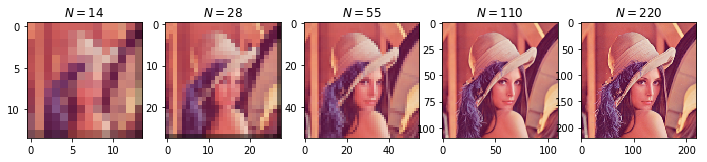The sampling of the colored imaged girl

The code defines N as the number of pixels per side. When N=14, that would mean that there are 14 pixels per image side. The same goes for the higher N values. As we compare all five images, the higher the N value is, the higher the image resolution is.

Let us move on to another part of the digital image. Quantization. This method controls the bit depth of (number of colors in layman's term) in the photo. Using the same colored image of a girl, we applied quantization to compare different bit depths.

`ks = 2**np.arange(1, 5)fig, ax = plt.subplots(1, len(ks), figsize=(12, 4))for i, k in enumerate(ks):    bins = np.linspace(0, lena.max(), k)    image = np.digitize(lena, bins)    image = np.vectorize(bins.tolist().__getitem__)(image-1).astype(int)    ax[i].imshow(image)    ax[i].set_title(r'\$k = %d\$' % k)`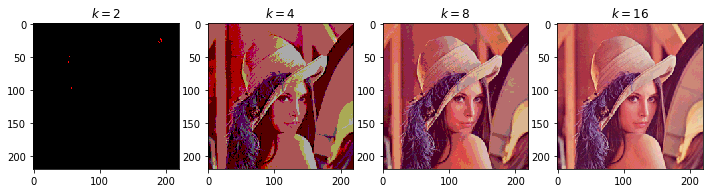quantization of the colored imaged girl

The code defines k as the bit depth. With k=2, the image has only two colors — red and black. Hence, those with higher k values have higher intensity and depth. If I were to print this photo, I would pick the image with k=16 for printing because it has the most depth.

In the last part of digital images, we tackle converting between image types. First, let us convert a colored image to grayscale. We have to import rgb2gray function from skimage library. Voila. The colored image has converted to gray scale.

`# Converting colored image to grayscalefrom skimage.color import rgb2graylena_gray = rgb2gray(lena)imshow(lena_gray);`

The colored image has converted to gray scale. We can further experiment by converting to a binary image. We have to also import the img_as_uint function from skimage library. This would allow us to apply thresholding on the pixel values. Those pixel values that are less than the threshold will be changed to the minimum allowed value while those above will replaced with the maximum allowed value. For this mage, we considered the mean of the converted gray scale as the threshold.

`# Converting grayscale image to binary imagefrom skimage import img_as_uint# for this demo, set threshold to average valuelena_binary = img_as_uint(lena_gray > lena_gray.mean())imshow(lena_binary);`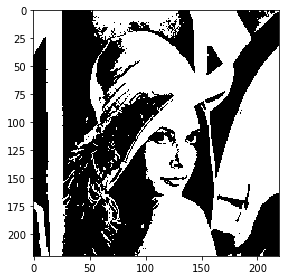converted to binary image from grayscale image

The resulting image looks like a pop art. Let’s making things more colorful by converting a grayscale image to colored image. We just need the gray2rgb function from skimage. color for conversion so that we can change the RGB value of the image.

`from skimage.color import gray2rgblena_color = gray2rgb(lena_gray)imshow(lena_color);fig, ax = plt.subplots(1, 3, figsize=(12,4))ax.imshow(lena[:,:,0], cmap='Reds')ax.set_title('Red')ax.imshow(lena[:,:,1], cmap='Greens')ax.set_title('Green')ax.imshow(lena[:,:,2], cmap='Blues')ax.set_title('Blue');`

The last coordinate decided the color channel. For the last conversion, we are converting the RGB images to Hue Saturation Value image and vice versa. For these two conversion, we would need to import rgb2hsv and hsv2rgb. We want to be able to see which colors are present in the image, how saturated the image is, and how bright is the image. And, the hsv version of the image will give that information.

`fig, ax = plt.subplots(1, 3, figsize=(12,4))ax.imshow(lena_hsv[:,:,0], cmap='hsv')ax.set_title('Hue')ax.imshow(lena_hsv[:,:,1], cmap='gray')ax.set_title('Saturation')ax.imshow(lena_hsv[:,:,2], cmap='gray')ax.set_title('Value');`
`from skimage.color import hsv2rgblena_rgb = hsv2rgb(lena_hsv)fig, ax = plt.subplots(1, 3, figsize=(12,4))ax.imshow(lena_rgb[:,:,0], cmap='Reds')ax.set_title('Red')ax.imshow(lena_rgb[:,:,1], cmap='Greens')ax.set_title('Green')ax.imshow(lena_rgb[:,:,2], cmap='Blues')ax.set_title('Blue');`